Simple Optimization with Python - PyData SG Meetup by Kai Xin @thiakx

In :
%matplotlib inline
import numpy as np
import pylab as pl
import seaborn as sns
import pulp
from scipy import stats
from scipy import optimize
from matplotlib import pyplot as plt
from matplotlib.path import Path
from matplotlib.patches import PathPatch
from mpl_toolkits.mplot3d import Axes3D
from matplotlib import cm
from matplotlib.colors import LogNorm


# Part I - 4 basic steps of framing an optimization problem.¶

Step1: Identify if problem is convex or non-convex.

In :
"""
Definition of a convex function
"""
x = np.linspace(-1, 2)

pl.figure(1, figsize=(5, 5))

# A convex function
pl.plot(x, x**2, linewidth=2)
pl.text(-.7, -.6**2, '$f$', size=20)

# The tangent in one point
pl.text(.1, -.75, "Convex problem, one optimal solution", size=15)
pl.text(0, -0.1, 'X', size=15)

# Convexity as barycenter
pl.ylim(ymin=-1)
pl.axis('off')
pl.tight_layout()

# Convexity as barycenter
pl.figure(2, figsize=(5, 5))
pl.clf()
pl.plot(x, x**2 + np.exp(-5*(x - .5)**2), linewidth=2)
pl.text(-.7, -.6**2, 'Non convex problem, many local minima and one global minimum', size=15)

pl.ylim(ymin=-1)
pl.axis('off')
pl.tight_layout()
pl.show()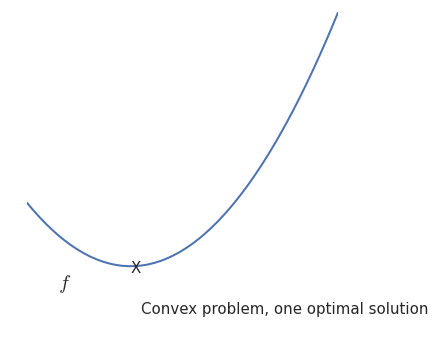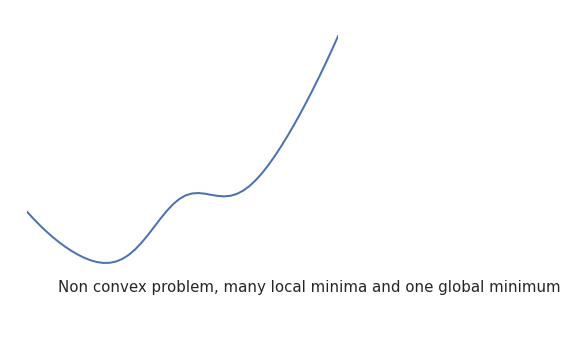Step2: Identify if problem is smooth or non-smooth.

In :
"""
Smooth vs non-smooth
"""
x = np.linspace(-1.5, 1.5, 101)

# A smooth function
pl.figure(1, figsize=(3, 2.5))
pl.clf()

pl.plot(x, np.sqrt(.2 + x**2), linewidth=2)
pl.text(-1, 0, 'Smooth functions are continuous and easier to solve', size=15)

pl.ylim(ymin=-.2)
pl.axis('off')
pl.tight_layout()

# A non-smooth function
pl.figure(2, figsize=(3, 5))
pl.clf()
#pl.plot(x, np.abs(x), linewidth=2)
pl.plot(x, linewidth=2)
pl.plot(x+1, linewidth=2)
pl.text(80, 0, 'Non smooth functions can be difficult or impossible to solve', size=15)

pl.ylim(ymin=-.2)
pl.axis('off')
pl.tight_layout()
pl.show()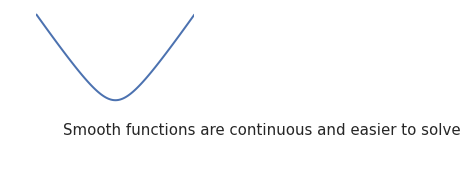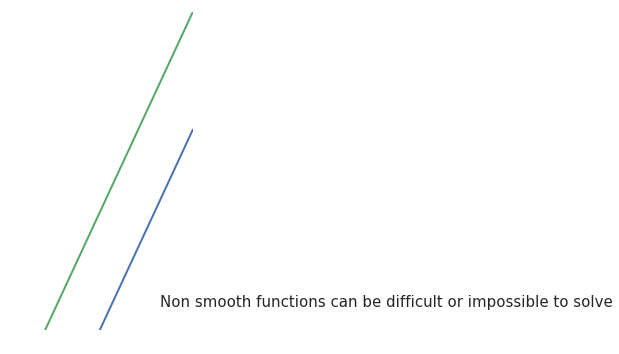Step3: Identify boundries / constraints.

In :
"""
Optimization with constraints
"""
x, y = np.mgrid[-2.9:5.8:.05, -2.5:5:.05]
x = x.T
y = y.T

for i in (1, 2):
# Create 2 figure: only the second one will have the optimization
# path
pl.figure(i, figsize=(3, 2.5))
pl.clf()
pl.axes([0, 0, 1, 1])

contours = pl.contour(np.sqrt((x - 3)**2 + (y - 2)**2),
extent=[-3, 6, -2.5, 5],
cmap=pl.cm.gnuplot)
pl.clabel(contours,
inline=1,
fmt='%1.1f',
fontsize=14)
pl.plot([-1.5, -1.5,  1.5,  1.5, -1.5],
[-1.5,  1.5,  1.5, -1.5, -1.5], 'k', linewidth=2)
pl.fill_between([ -1.5,  1.5],
[ -1.5, -1.5],
[  1.5,  1.5],
color='.8')
pl.axvline(0, color='k')
pl.axhline(0, color='k')

pl.text(-.9, 4.4, '$x_2$', size=20)
pl.text(5.6, -.6, '$x_1$', size=20)
pl.axis('equal')
pl.axis('off')

# And now plot the optimization path
accumulator = list()

def f(x):
# Store the list of function calls
accumulator.append(x)
return np.sqrt((x - 3)**2 + (x - 2)**2)

# We don't use the gradient, as with the gradient, L-BFGS is too fast,
# and finds the optimum without showing us a pretty path
def f_prime(x):
r = np.sqrt((x - 3)**2 + (x - 2)**2)
return np.array(((x - 3)/r, (x - 2)/r))

print "Minimum found at", optimize.fmin_l_bfgs_b(f, np.array([0, 0]), approx_grad=1,bounds=((-1.5, 1.5), (-1.5, 1.5)))

accumulated = np.array(accumulator)
pl.plot(accumulated[:, 0], accumulated[:, 1])

pl.show()

Minimum found at (array([ 1.5,  1.5]), 1.5811388300841898, {'warnflag': 0, 'task': 'CONVERGENCE: NORM_OF_PROJECTED_GRADIENT_<=_PGTOL', 'grad': array([-0.94868331, -0.31622778]), 'nit': 2, 'funcalls': 3})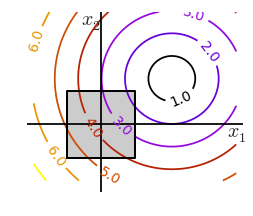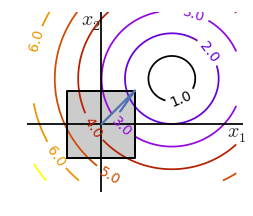Step4: Determine the size of data. Some optimizing solvers won't work on too large / too small data.# Part II - Simple Optimization Problem¶

Giapetto’s Woodcarving, Inc., manufactures two types of wooden toys: soldiers and trains.

• A soldier sells for 27 dollars and uses 10 dollars worth of raw materials. Each soldier that is manufactured increases Giapetto’s variable labor and overhead costs by 14 dollars.

• A train sells for 21 dollars and uses 9 dollars worth of raw materials. Each train built increases Giapetto’s variable labor and overhead costs by 10 dollars.

• The manufacture of wooden soldiers and trains requires two types of skilled labor: carpentry and finishing. A soldier requires 2 hours of finishing labor and 1 hour of carpentry labor. A train requires 1 hour of finishing labor and 1 hour of carpentry labor.

• Each week, Giapetto can obtain all the needed raw material but only 100 finishing hours and 80 carpentry hours. Demand for trains is unlimited, but at most 40 soldiers are bought each week. Giapetto wants to maximize weekly profit (revenues-costs).

• Formulate a mathematical model of Giapetto’s situation that can be used to maximize Giapetto’s weekly profit.

So here we basically have a convex, smooth problem with clear constraints and small data.

In :
# use seaborn to change the default graphics to something nicer
# and set a nice color palette
sns.set_palette("Set1", 8)

# create the plot object
fig, ax = plt.subplots(figsize=(8, 8))
s = np.linspace(0, 100)

# add carpentry constraint: trains + soldiers <= 80
plt.plot(s, 80 - s, lw=3, label='carpentry')
#plt.fill_between(s, 0, 80 - s, alpha=0.1)

# add finishing constraint: trains + 2*soldiers <= 100
plt.plot(s, 100 - 2 * s, lw=3, label='finishing')
#plt.fill_between(s, 0, 100 - 2 * s, alpha=0.1)

# add demains constraint: soldiers <= 40
plt.plot(40 * np.ones_like(s), s, lw=3, label='demand')
#plt.fill_betweenx(s, 0, 40, alpha=0.1)

plt.plot(np.zeros_like(s), s, lw=3, label='t non-negative')
plt.plot(s, np.zeros_like(s), lw=3, label='s non-negative')

# highlight the feasible region
path = Path([
(0., 0.),
(0., 80.),
(20., 60.),
(40., 20.),
(40., 0.),
(0., 0.),
])
patch = PathPatch(path, label='feasible region', alpha=0.5)

# labels and stuff
plt.xlabel('soldiers', fontsize=16)
plt.ylabel('trains', fontsize=16)
plt.xlim(-0.5, 100)
plt.ylim(-0.5, 100)
plt.legend(fontsize=14)
plt.show()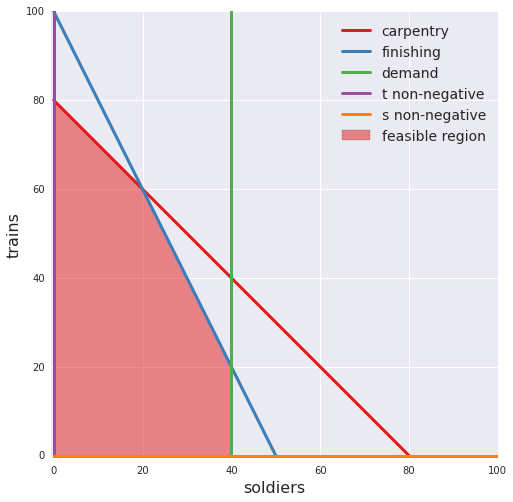In :
# create the LP object, set up as a maximization problem
prob = pulp.LpProblem('Giapetto', pulp.LpMaximize)

# set up decision variables
soldiers = pulp.LpVariable('soldiers', lowBound=0, cat='Integer')
trains = pulp.LpVariable('trains', lowBound=0, cat='Integer')

# model weekly production costs
raw_material_costs = 10 * soldiers + 9 * trains
variable_costs = 14 * soldiers + 10 * trains

# model weekly revenues from toy sales
revenues = 27 * soldiers + 21 * trains

# use weekly profit as the objective function to maximize
profit = revenues - (raw_material_costs + variable_costs)
prob += profit  # here's where we actually add it to the obj function

# add constraints for available labor hours
carpentry_hours = soldiers + trains
prob += (carpentry_hours <= 80)

finishing_hours = 2*soldiers + trains
prob += (finishing_hours <= 100)

# add constraint representing demand for soldiers
prob += (soldiers <= 40)

print(prob)

Giapetto:
MAXIMIZE
3*soldiers + 2*trains + 0
SUBJECT TO
_C1: soldiers + trains <= 80

_C2: 2 soldiers + trains <= 100

_C3: soldiers <= 40

VARIABLES
0 <= soldiers Integer
0 <= trains Integer


In :
# solve the LP using the default solver
optimization_result = prob.solve()

# make sure we got an optimal solution
assert optimization_result == pulp.LpStatusOptimal

# display the results
for var in (soldiers, trains):
print('Optimal weekly number of {} to produce: {:1.0f}'.format(var.name, var.value()))

Optimal weekly number of soldiers to produce: 20
Optimal weekly number of trains to produce: 60

In :
sns.set_palette("Set1", 8)

fig, ax = plt.subplots(figsize=(9, 8))
s = np.linspace(0, 100)

# plot the constraints again
plt.plot(s, 80 - s, lw=3, label='carpentry')
plt.plot(s, 100 - 2 * s, lw=3, label='finishing')
plt.plot(40 * np.ones_like(s), s, lw=3, label='demand')
plt.plot(np.zeros_like(s), s, lw=3, label='t non-negative')
plt.plot(s, np.zeros_like(s), lw=3, label='s non-negative')

# plot the possible (s, t) pairs
pairs = [(s, t) for s in np.arange(101)
for t in np.arange(101)
if (s + t) <= 80
and (2 * s + t) <= 100
and s <= 40]

# split these into our variables
ss, ts = np.hsplit(np.array(pairs), 2)

# caculate the objective function at each pair
z = 3*ss + 2*ts  # the objective function

# plot the results
plt.scatter(ss, ts, c=z, cmap=plt.cm.get_cmap('RdYlBu_r'), label='profit', zorder=3)

# labels and stuff
cb = plt.colorbar()
cb.set_label('profit', fontsize=14)
plt.xlabel('soldiers', fontsize=16)
plt.ylabel('trains', fontsize=16)
plt.xlim(-0.5, 100)
plt.ylim(-0.5, 100)
plt.legend(fontsize=14)
plt.show()

plt.show()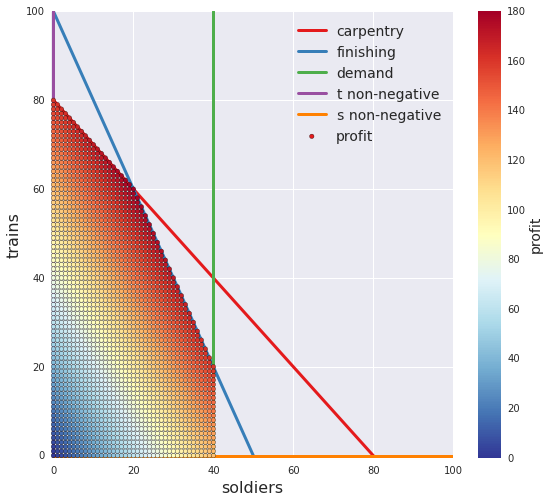# Part III - How to select a solver¶

Solving the famous Rosenbrock Banana. Global minimum at 1,1 with 0 value. Reference: http://scipy-lectures.github.io/advanced/mathematical_optimization/

In :
fig = plt.figure()
ax = Axes3D(fig, azim = -128, elev = 43)
s = .05
X = np.arange(-2, 2.+s, s)
Y = np.arange(-1, 3.+s, s)
X, Y = np.meshgrid(X, Y)
Z = (1.-X)**2 + 100.*(Y-X*X)**2
ax.plot_surface(X, Y, Z, rstride = 1, cstride = 1, norm = LogNorm(), cmap = cm.jet)

plt.xlabel("x")
plt.ylabel("y")
plt.show()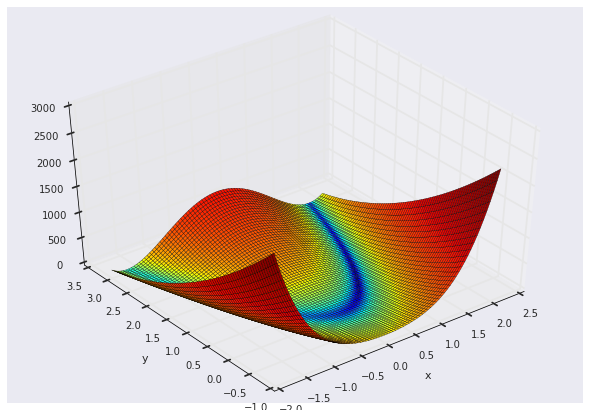In :
def f(x): return (1 - x)**2 + 100*(x - x**2)**2 # The rosenbrock function
print "BruteForce:",optimize.brute(f, ((-1, 2), (-1, 2)))
print "anneal:",optimize.anneal(f, [2, 2]) #simulated annealing
print "anneal:",optimize.anneal(f, [2, 2],maxiter=10000,schedule='boltzmann', learn_rate=0.5,upper=2,lower=-1)

BruteForce: [ 0.99998188  0.99996297]
anneal:Warning: Cooled to 174747.925213 at [  -47.50619239  2215.31782985] but this is not the smallest point found.
(array([ -17.94360667,  321.7192422 ]), 5)
anneal:Warning: Maximum number of iterations exceeded.
(array([ 1.00676758,  1.01542639]), 3)

In :
#other optimization solvers
print optimize.fmin_cg(f, [2, 2]) #Conjugate gradient descent
print optimize.fmin(f, [2, 2]) #Simplex method: the Nelder-Mead
print optimize.fmin_powell(f, [2, 2]) #the Powell algorithm: An efficient method for finding the minimum of a function of several variables without calculating derivatives

Warning: Desired error not necessarily achieved due to precision loss.
Current function value: 10.321787
Iterations: 1
Function evaluations: 40
[ 1.3569372   2.16056499]
Optimization terminated successfully.
Current function value: 0.000000
Iterations: 62
Function evaluations: 119
[ 0.99998292  0.99996512]
Optimization terminated successfully.
Current function value: 0.000000
Iterations: 12
Function evaluations: 339
[ 1.  1.]